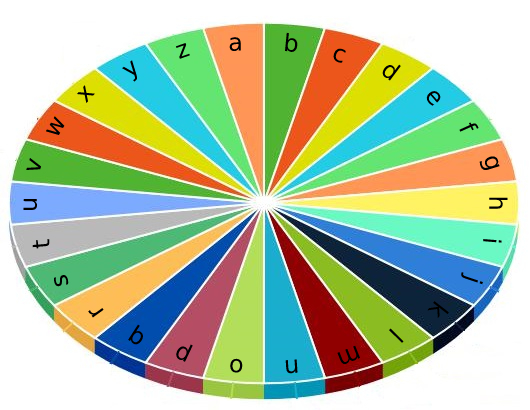1974. Minimum Time to Type Word Using Special Typewriter

There is a special typewriter with lowercase English letters `'a'` to `'z'` arranged in a circle with a pointer. A character can only be typed if the pointer is pointing to that character. The pointer is initially pointing to the character `'a'`.Each second, you may perform one of the following operations:

• Move the pointer one character counterclockwise or clockwise.
• Type the character the pointer is currently on.

Given a string `word`, return the minimum number of seconds to type out the characters in `word`.

Example 1:

```Input: word = "abc"
Output: 5
Explanation:
The characters are printed as follows:
- Type the character 'a' in 1 second since the pointer is initially on 'a'.
- Move the pointer clockwise to 'b' in 1 second.
- Type the character 'b' in 1 second.
- Move the pointer clockwise to 'c' in 1 second.
- Type the character 'c' in 1 second.
```

Example 2:

```Input: word = "bza"
Output: 7
Explanation:
The characters are printed as follows:
- Move the pointer clockwise to 'b' in 1 second.
- Type the character 'b' in 1 second.
- Move the pointer counterclockwise to 'z' in 2 seconds.
- Type the character 'z' in 1 second.
- Move the pointer clockwise to 'a' in 1 second.
- Type the character 'a' in 1 second.
```

Example 3:

```Input: word = "zjpc"
Output: 34
Explanation:
The characters are printed as follows:
- Move the pointer counterclockwise to 'z' in 1 second.
- Type the character 'z' in 1 second.
- Move the pointer clockwise to 'j' in 10 seconds.
- Type the character 'j' in 1 second.
- Move the pointer clockwise to 'p' in 6 seconds.
- Type the character 'p' in 1 second.
- Move the pointer counterclockwise to 'c' in 13 seconds.
- Type the character 'c' in 1 second.
```

Constraints:

• `1 <= word.length <= 100`
• `word` consists of lowercase English letters.

1974. Minimum Time to Type Word Using Special Typewriter
``````struct Solution;

impl Solution {
fn min_time_to_type(word: String) -> i32 {
let mut current = b'a';
let mut res = 0;
for b in word.bytes() {
if b == current {
res += 1;
} else {
let d = if b > current {
b - current
} else {
current - b
};
let d = d.min(26 - d);
current = b;
res += d as i32 + 1;
}
}
res
}
}

#[test]
fn test() {
let word = "abc".to_string();
let res = 5;
assert_eq!(Solution::min_time_to_type(word), res);
let word = "bza".to_string();
let res = 7;
assert_eq!(Solution::min_time_to_type(word), res);
let word = "zjpc".to_string();
let res = 34;
assert_eq!(Solution::min_time_to_type(word), res);
}
``````